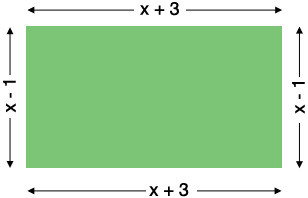SEARCH HOMEMath Central Quandaries & QueriesQuestion from Imran, a student: The length of a rectangle is x+3 centimeters. The width of the rectangle is x-1 centimeters. Find an expression in terms of x for the perimeter of the rectangle. Give your expressions in it's simplest form. Thank You.Hi,

I drew a diagram.The perimeter is the distance all the way around, so it is the sum of the lengths of the four sides. Write this sum and simplify it.

PennyMath Central is supported by the University of Regina and the Imperial Oil Foundation.